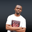Related Tags

php
value
datatype
communitycreator

# How to check if a defined variable is null in PHPNDUKWE CHIDERA K.

Different variables are given values when they are declared. PHP uses these values to determine the data type of the variables.

The PHP programming language can identify a number of data types.

### The null data type

When you want to create a new variable, your program code tells your computer to leave a memory space that attaches the address of the memory space to the name of the variable. This causes the variable name to point to an address in memory where the stored data can be found.

If you declare a variable and don’t give it a value like the one below, the variable is automatically given a null value.

$myTravelLog;  null describes the value of any variable that doesn’t have value. Essentially, null means no value. Note: Being null does not mean empty. You will see this difference in the snippet below. ### How to check if a defined variable is null To check if a defined variable is null, we can use two different methods. ### 1. The isset() method The isset() method is mainly used to check for null variables, especially when collecting form data. This helps us avoid keeping non-nullable columns without data. Let’s take a look at the code snippet to see how this is done. #### Code <?php$x = 0;
$plays = ""; if(isset($x) && isset($plays)){ echo "These are empty not null <br>"; //zero is empty in the context of integers } if(empty($x) && empty($plays)){ echo "These are empty <br>"; // will show us that zero as integer is seen as empty }$jan;
if(isset($jan)){ echo "This is null <br>"; /* This will return false so our echo won't display because variable$jan was not given a value */

}
$tangle = null; if(isset($tangle)){
echo "This is declared as null <br>";
}
/* This also returns false, because with a null value
variable $tangle has no value*/ ?> Sample isset() code #### Explanation In this snippet we can see how the isset() function returns false in situations where the variable is null. It returns true when the variable is something else, even if it is empty. When validating your form input, try to check for empty as well. Don’t just end validation with isset(). ### 2. The is_null() method The is_null() method is an inbuilt PHP function which that verifies whether or not a variable is null. This method is supported by PHP 4 and later versions. Unlike the isset() method, the is_null method returns the following when we use it to check the variable: • Returns true if the variable is null. • Returns false if the variable is not null. Let’s look at some code. #### Code <?php$a_null_value;
$another_null = null;$noneNull = "I have a value.";
$thisIsEmpty = ''; if(is_null($a_null_value)){
//will echo "just null" if it returns
//true else nothing
echo "just null <br>";
}
if(is_null($another_null)){ //will echo "just another null" if it returns //true else nothing echo "just another null <br>"; } if(is_null($noneNull)){
//will echo the indicated string if it returns
//true else nothing
echo "No display cuz this is a string ".$noneNull."<br>"; } if(!is_null($thisIsEmpty)){
//This will return true because the variable is an
//empty string variable and will display the echo
echo "will display because ".\$thisIsEmpty." is empty";
}

?>
Example use of the is_null() function

#### Explanation

The code above is an example of how to use the is_null() function to check if a variable is nullmeaning that it has no declared value.

The different if functions used in the snippet above check the different variables and return the necessary value according to the value of the variables.

RELATED TAGS

php
value
datatype
communitycreator

CONTRIBUTORNDUKWE CHIDERA K.
RELATED COURSES

View all Courses

Keep Exploring

Learn in-demand tech skills in half the time